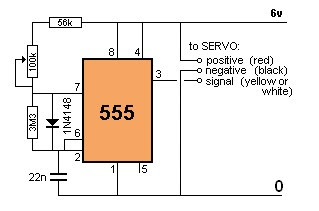Sunday, June 16, 2013

Electronic Circuit Schematic of Servo Motor Controller using IC NE555

Robometricschool. - One motor kind that many used in robot, UAV, etc is Servo Motor. In this time we want to show you one kinds of controller of Servo motor using IC NE555 electronic circuit schematic.Figure 1. Servo Motor

You will find there are many ways how to control servo motor this days. We here will show very simple circuit schematic of servo motor controller using IC NE 555 as follow.

Servo Motor Controller Schematic CircuitFigure 2. Servo Motor Controller Schematic using NE555

Component List

The main component of the Electronic Circuit Schematic of Servo Motor Controller here is IC NE 555. And for all component you can see easily from figure 2 above.

Schematic Performance

Servo motor controller circuit in the figure 2 above is an example of a simple servo motor controller. This servo motor controller circuit using IC NE555 pulse generator controls the servo motor rotation.

Setting of IC NE555 pulse generator is working astabil-multivibrator with a frequency of 50 Hz with a pulse period of 20 ms and positive pulse width (duty cycle) can be set. This servo motor controller circuit can be operated using a voltage source + 6 volts DC. Setting motion servo motor with servo motor control circuit is done by adjusting the potentio-meter lever 100 Kohm as like in figure 2 above.

Here are the performance of the servo motor controller above:
1. Servo motor control output path is taken from pin 3 IC NE555 and connected to the signal pin servo motors.
2. This control signal will control the movement of the servo motors are regulated by a servo motor control circuit through a 100 Kohm potentiometer on the circuit.
3. 100 Kohm potentiometer on the controller circuit above will set the positive pulse period to control servo motors from 0.9 ms to 2.1 ms.
4. With a positive variation of pulse width from 0.9 to 2.1 ms the servo motor can then set conditions for stopping the movement of the moving clockwise, stopping in the middle position and moving counter-clockwise.
Source: SkemarangkaianPCB